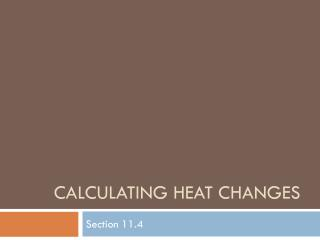DownloadDownload PresentationCalculating heat changes

# Calculating heat changes

Download Presentation## Calculating heat changes

- - - - - - - - - - - - - - - - - - - - - - - - - - - E N D - - - - - - - - - - - - - - - - - - - - - - - - - - -
##### Presentation Transcript

1. Calculating heat changes Section 11.4

2. After reading Section 11.4, you should know: • What Hess’s Law is • How to apply Hess’s Law to a chemical equation • How to calculate the heat of formation for a given chemical equation

3. Hess’s Law • The heat of 2 reactions added together will equal the heat of a 3rd reaction Adding 2 equations together…you can cancel compounds out IF they are exactly the same on the reactants and products side 2Al + 11/2 O2 --> Al2O3  H = -1669.8 kJ Fe2O3 --> 2Fe + 11/2 O2  H = 824.2 kJ 2Al + Fe2O3 --> 2Fe + Al2O3  H = -844.8 kJ

4. 0 Hf =standard heat of formation Change in enthalpy when 1 mol of new compounds form under standard conditions Table of values on page 316 Ex: Calculate theH0for 2NO(g) + O2 --> 2NO2 Hf NO = 90.37 kJ / mol Hf O2 = 0.0 kJ / mol Hf NO2 = 33.85 kJ / mol 0 0 0

5. 0 1. Find sum ofHf reactants Remember, you have 2 moles of NO 0 2. Find sum ofHf products 3. Find the difference between Hf products and Hf reactants You should have gotten: -113.04 kJ / mol

6. After reading Section 11.4, you should know: • What Hess’s Law is • How to apply Hess’s Law to a chemical equation • How to calculate the heat of formation for a given chemical equation